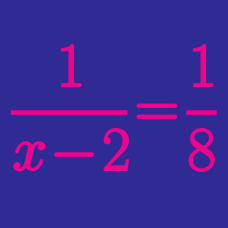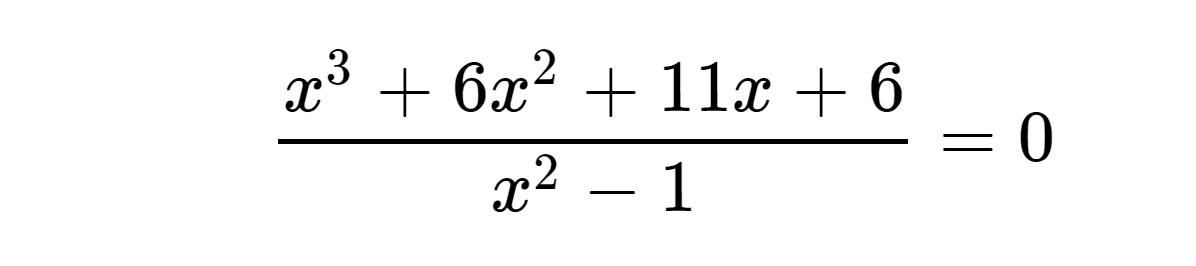Algebra

# Rational Functions Problem SolvingHow many real values of $x$ satisfy the above equation?

$f$ and $g$ are functions defined as $f(x) = 16 + \frac{9}{x}$ and $g(x) = \frac{9}{x}$. What is the value of $(f+g)(18)$?

What is the nearest integer value of $y=\frac{6x-23}{x-5}$ when $x=10000$?

$f$ and $g$ are functions defined as $f(x)= 5x + \frac{6}{2x-2}$ and $g(x) = \frac{4x}{3x-2}$. What is the value of $\left(f\circ g\right)(4)$?

Note: $f \circ g$ denotes the composition of the 2 functions.

If the asymptotes of the graph $y=\frac{7x+2}{x-2a}$ are $x=24$ and $y=b$, what is the value of $ab$?

×